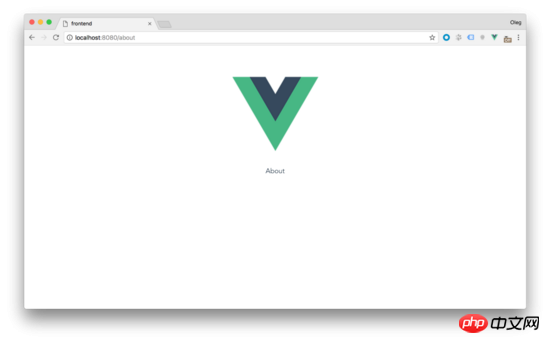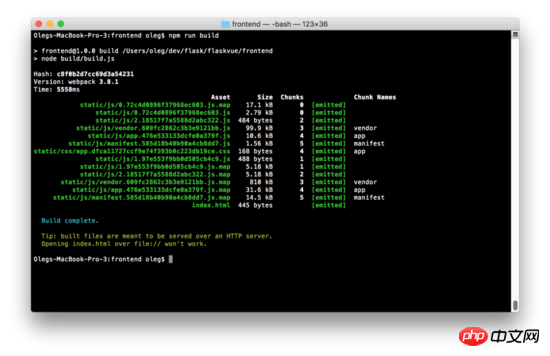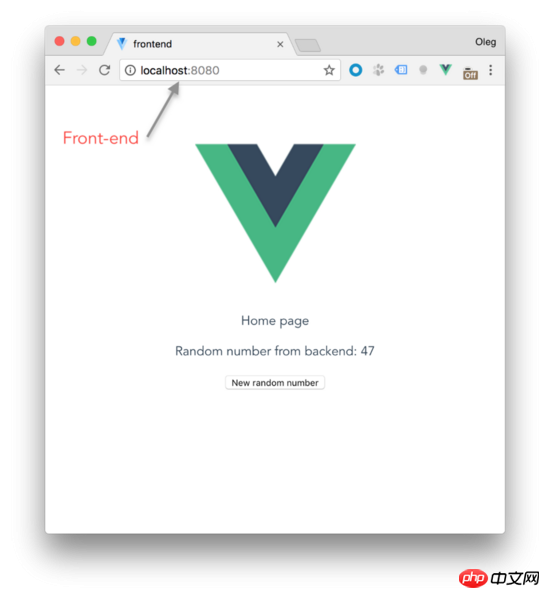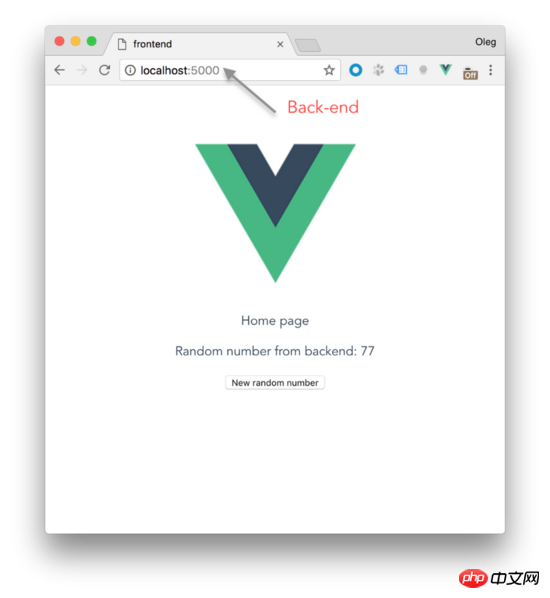Flask为 index.html 服务， index.html 包含我的vue.js App。

`\$ npm install -g vue-cli`

```\$ mkdir flaskvue
\$ vue init webpack frontend```

Vue 只在运行时构建。

ok，接着来：

```\$ cd frontend
\$ npm install
# after installation
\$ npm run dev```

```// Home.vue
<template>
<p>
</p>
</template>```

and

```// About.vue
<template>
<p>
</p>
</template>```

```import Vue from 'vue'
import Router from 'vue-router'
const routerOptions = [
{ path: '/', component: 'Home' },
]
const routes = routerOptions.map(route => {
return {
...route,
component: () => import(`@/components/\${route.component}.vue`)
}
})
Vue.use(Router)
export default new Router({
routes,
mode: 'history'
})``````index: path.resolve(dirname, '../dist/index.html'),
assetsRoot: path.resolve(dirname, '../dist'),```

```index: path.resolve(dirname, '../../dist/index.html'),
assetsRoot: path.resolve(dirname, '../../dist'),``````\$ mkdir backend
\$ cd backend
\$ virtualenv -p python3 venv```

`\$ source venv/bin/activate`

`(venv) pip install Flask`

```(venv) cd ..
(venv) touch run.py```

```from flask import Flask, render_template
static_folder = "./dist/static",
template_folder = "./dist")
@app.route('/')
def index():
return render_template("index.html")```

`(venv) FLASK_APP=run.py FLASK_DEBUG=1 flask run`

```@app.route('/', defaults={'path': ''})
@app.route('/<path:path>')
def catch_all(path):
return render_template("index.html")```

```const routerOptions = [
{ path: '/', component: 'Home' },
{ path: '*', component: 'NotFound' }
]```

```// NotFound.vue
<template>
<p>
</p>
</template>```

```from flask import Flask, render_template, jsonify
from random import *
static_folder = "./dist/static",
template_folder = "./dist")
@app.route('/api/random')
def random_number():
response = {
'randomNumber': randint(1, 100)
}
return jsonify(response)
@app.route('/', defaults={'path': ''})
@app.route('/<path:path>')
def catch_all(path):
return render_template("index.html")```

```{
"randomNumber": 36
}```

```<template>
<p>
<p>Random number from backend: {{ randomNumber }}</p>
<button @click="getRandom">New random number</button>
</p>
</template>
<script>
export default {
data () {
return {
randomNumber: 0
}
},
methods: {
getRandomInt (min, max) {
min = Math.ceil(min)
max = Math.floor(max)
return Math.floor(Math.random() * (max - min + 1)) + min
},
getRandom () {
this.randomNumber = this.getRandomInt(1, 100)
}
},
created () {
this.getRandom()
}
}
</script>```

1. 在初始化变量 randomNumber 等于0。

2. 在methods部分我们通过 getRandomInt(min, max) 功能来从指定的范围内返回一个随机数， getrandom 函数将生成随机数并将赋值给 randomNumber

3. 组件方法 getrandom 创建后将会被调用来初始化随机数

4. 在按钮的单击事件我们将用 getrandom 方法得到新的随机数

```(venv) cd frontend
(venv) npm install --save axios```

```import axios from 'axios'
methods: {
getRandom () {
// this.randomNumber = this.getRandomInt(1, 100)
this.randomNumber = this.getRandomFromBackend()
},
getRandomFromBackend () {
const path = `http://localhost:5000/api/random`
axios.get(path)
.then(response => {
this.randomNumber = response.data.randomNumber
})
.catch(error => {
console.log(error)
})
}
}```

`(venv) pip install -U flask-cors`

```from flask_cors import CORS
static_folder = "./dist/static",
template_folder = "./dist")
cors = CORS(app, resources={r"/api#zs#": {"origins": "*"}})```

```import requests
@app.route('/', defaults={'path': ''})
@app.route('/<path:path>')
def catch_all(path):
if app.debug:
return requests.get('http://localhost:8080/{}'.format(path)).text
return render_template("index.html")```### 吐槽小黑屋()

* 这里是“吐槽小黑屋”，所有人可看，只保留当天信息。

• ###Erlo.vip2021-01-22 13:37:27Hello、欢迎使用吐槽小黑屋，这就是个吐槽的地方。
• 返回顶部### 给这篇文章打个标签吧~

棒极了 糟糕透顶 好文章 PHP JAVA JS 小程序 Python SEO MySql 确认